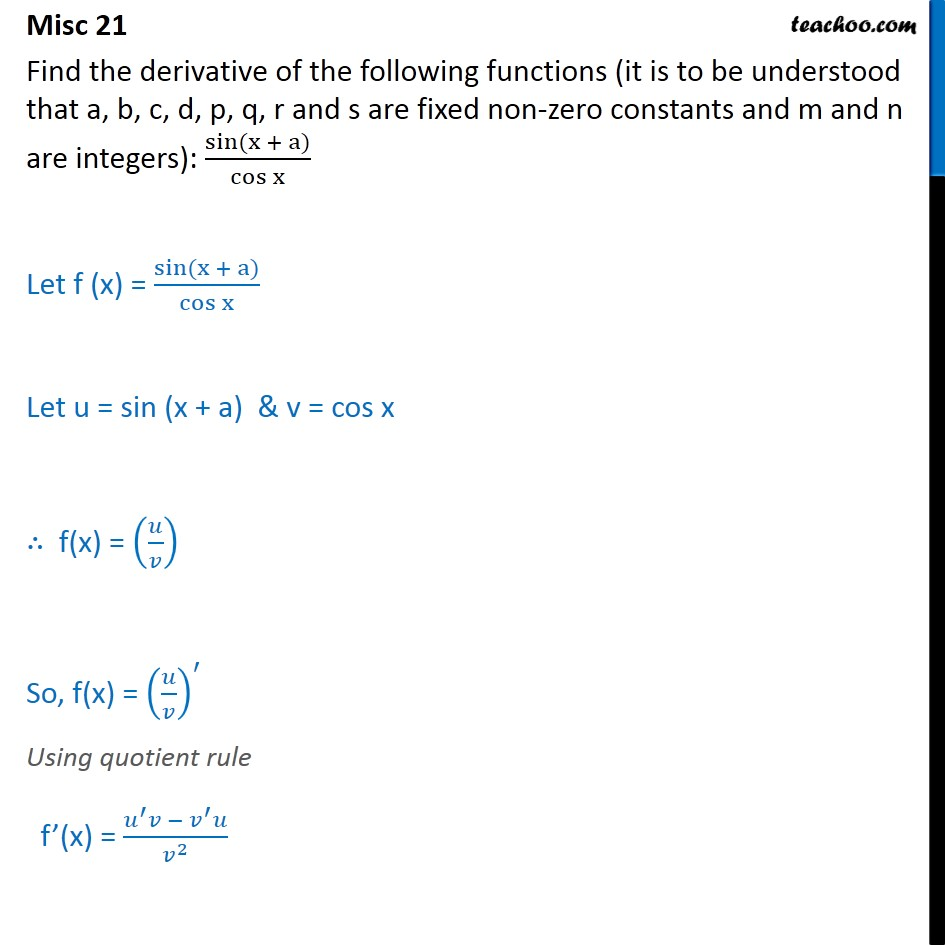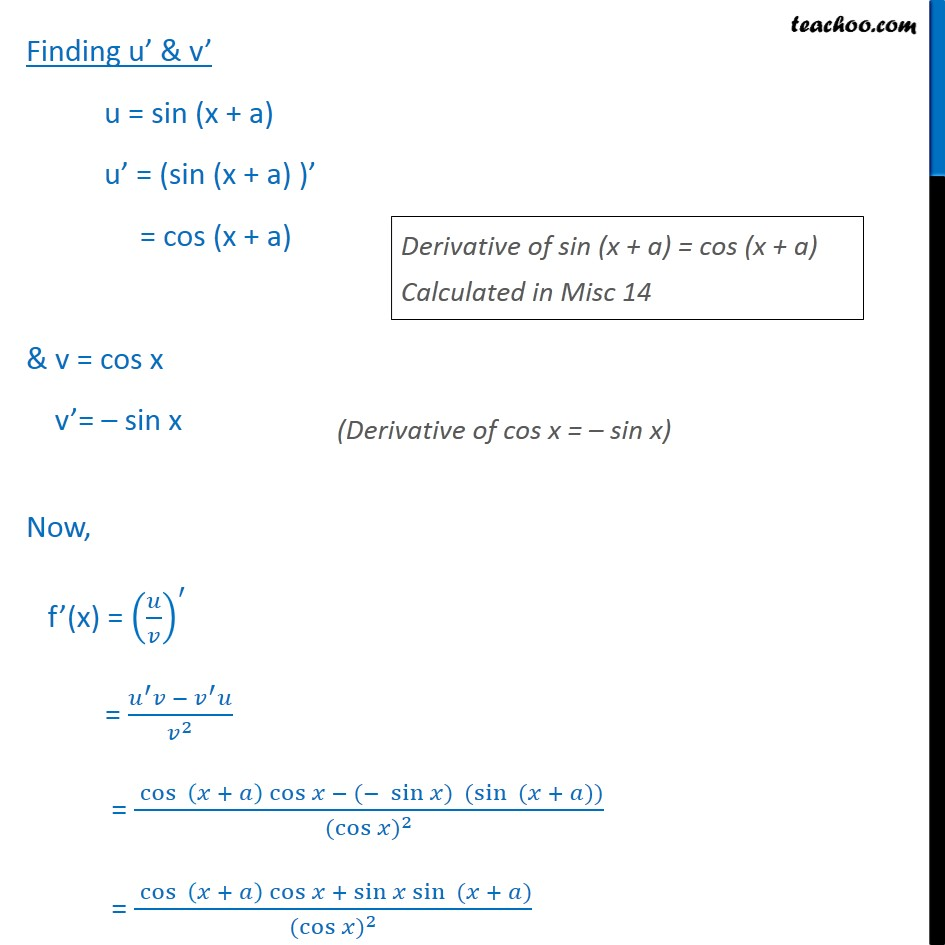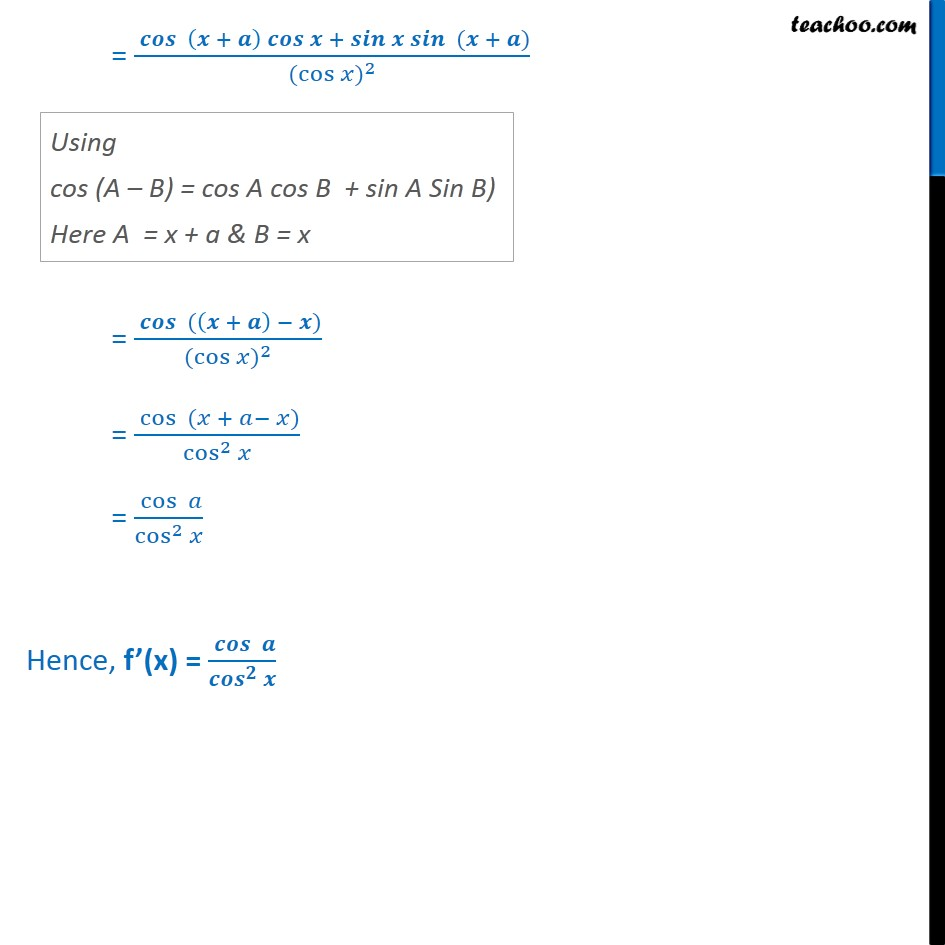Derivatives by formula - sin & cos

Chapter 12 Class 11 Limits and Derivatives
Concept wiseLearn in your speed, with individual attention - Teachoo Maths 1-on-1 Class

### Transcript

Misc 21 Find the derivative of the following functions (it is to be understood that a, b, c, d, p, q, r and s are fixed non-zero constants and m and n are integers): sin (x + a) cos x Let f (x) = sin (x + a) cos x Let u = sin (x + a) & v = cos x f(x) = So, f(x) = Using quotient rule f (x) = 2 Finding u & v u = sin (x + a) u = (sin (x + a) ) = cos (x + a) & v = cos x v = sin x Now, f (x) = = 2 = cos + cos ( sin ) (sin ( + )) ( cos ) 2 = cos + cos + sin sin ( + ) ( cos ) 2 = + + ( + ) ( cos ) 2 = ( + ) ( cos ) 2 = cos ( + ) cos 2 = cos cos 2 Hence, f (x) =